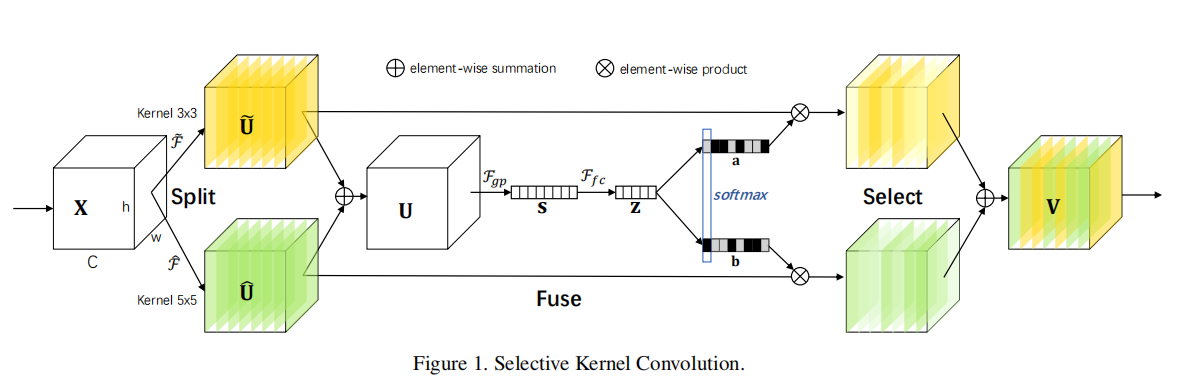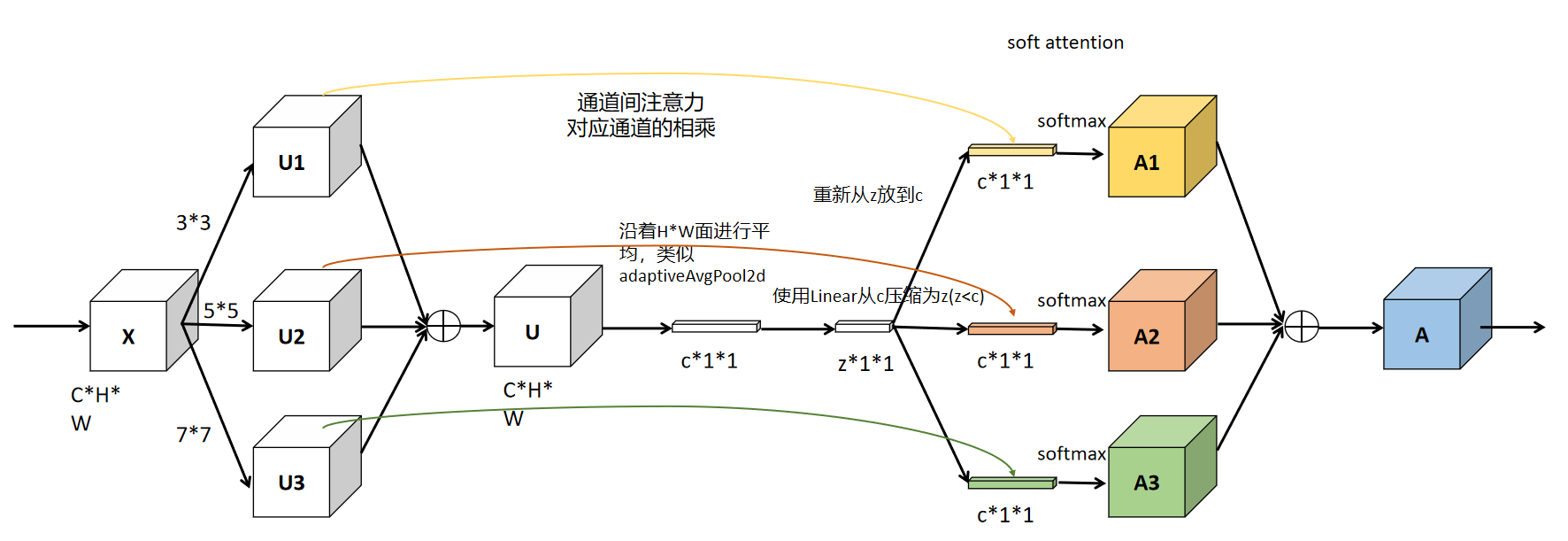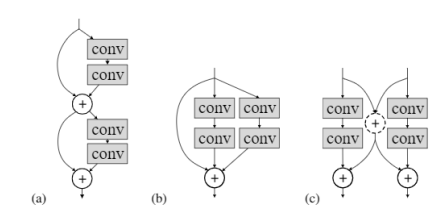# 【CV中的Attention机制】Selective-Kernel-Networks-SE进化版

## 1. SKNet¶

SKNet是SENet的加强版，结合了SE opetator、Merge-and-Run Mappings以及attention on inception block的产物。其最终提出的也是与SE类似的一个模块，名为SK模块, 可以自适应调节自身的感受野。

SK模块核心思想就是：用multiple scale feature汇总的information来channel-wise地指导如何分配侧重使用哪个kernel的表征

-- 李翔• 从C线性变换为Z维，再到C维度，这个部分与SE模块的实现是一致的。
• 多分支的操作借鉴自：inception。
• 整个流程类似merge-and-run mapping。## 2. 代码实现¶

import torch.nn as nn
import torch

class SKConv(nn.Module):
def __init__(self, features, WH, M, G, r, stride=1, L=32):
super(SKConv, self).__init__()
d = max(int(features / r), L)
self.M = M
self.features = features
self.convs = nn.ModuleList([])
for i in range(M):
# 使用不同kernel size的卷积
self.convs.append(
nn.Sequential(
nn.Conv2d(features,
features,
kernel_size=3 + i * 2,
stride=stride,
groups=G), nn.BatchNorm2d(features),
nn.ReLU(inplace=False)))

self.fc = nn.Linear(features, d)
self.fcs = nn.ModuleList([])
for i in range(M):
self.fcs.append(nn.Linear(d, features))
self.softmax = nn.Softmax(dim=1)

def forward(self, x):
for i, conv in enumerate(self.convs):
fea = conv(x).unsqueeze_(dim=1)
if i == 0:
feas = fea
else:
feas = torch.cat([feas, fea], dim=1)
fea_U = torch.sum(feas, dim=1)
fea_s = fea_U.mean(-1).mean(-1)
fea_z = self.fc(fea_s)
for i, fc in enumerate(self.fcs):
print(i, fea_z.shape)
vector = fc(fea_z).unsqueeze_(dim=1)
print(i, vector.shape)
if i == 0:
attention_vectors = vector
else:
attention_vectors = torch.cat([attention_vectors, vector],
dim=1)
attention_vectors = self.softmax(attention_vectors)
attention_vectors = attention_vectors.unsqueeze(-1).unsqueeze(-1)
fea_v = (feas * attention_vectors).sum(dim=1)
return fea_v

if __name__ == "__main__":
t = torch.ones((32, 256, 24,24))
sk = SKConv(256,WH=1,M=2,G=1,r=2)
out = sk(t)
print(out.shape)


## 3. 参考¶

sknet论文地址：https://arxiv.org/pdf/1903.06586.pdf

merge and run mapping: https://arxiv.org/pdf/1611.07718.pdf